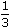# Aptitude - Simple Interest

### Exercise :: Simple Interest - General Questions

6.

A sum of Rs. 12,500 amounts to Rs. 15,500 in 4 years at the rate of simple interest. What is the rate of interest?

 A. 3% B. 4% C. 5% D. 6% E. None of these

Explanation:

S.I. = Rs. (15500 - 12500) = Rs. 3000.

 Rate =100 x 3000% = 6% 12500 x 4

Video Explanation: https://youtu.be/SIcQu1HOmOk

7.

An automobile financier claims to be lending money at simple interest, but he includes the interest every six months for calculating the principal. If he is charging an interest of 10%, the effective rate of interest becomes:

 A. 10% B. 10.25% C. 10.5% D. None of these

Explanation:

Let the sum be Rs. 100. Then,

 S.I. for first 6 months = Rs.100 x 10 x 1= Rs. 5 100 x 2

 S.I. for last 6 months = Rs.105 x 10 x 1= Rs. 5.25 100 x 2

So, amount at the end of 1 year = Rs. (100 + 5 + 5.25) = Rs. 110.25Effective rate = (110.25 - 100) = 10.25%

8.

A lent Rs. 5000 to B for 2 years and Rs. 3000 to C for 4 years on simple interest at the same rate of interest and received Rs. 2200 in all from both of them as interest. The rate of interest per annum is:

A. 5%
B. 7%
C.
 7 1 % 8
D. 10%

Explanation:

Let the rate be R% p.a.

 Then,5000 x R x 2+3000 x R x 4= 2200. 100 100100R + 120R = 2200R =2200= 10. 220Rate = 10%.

9.

A sum of Rs. 725 is lent in the beginning of a year at a certain rate of interest. After 8 months, a sum of Rs. 362.50 more is lent but at the rate twice the former. At the end of the year, Rs. 33.50 is earned as interest from both the loans. What was the original rate of interest?

 A. 3.6% B. 4.5% C. 5% D. 6% E. None of these

Explanation:

Let the original rate be R%. Then, new rate = (2R)%.

Note:
Here, original rate is for 1 year(s); the new rate is for only 4 months i.e.year(s).725 x R x 1+362.50 x 2R x 1= 33.50 100 100 x 3(2175 + 725) R = 33.50 x 100 x 3(2175 + 725) R = 10050(2900)R = 10050R = 10050 = 3.46 2900Original rate = 3.46%

10.

A man took loan from a bank at the rate of 12% p.a. simple interest. After 3 years he had to pay Rs. 5400 interest only for the period. The principal amount borrowed by him was:

 A. Rs. 2000 B. Rs. 10,000 C. Rs. 15,000 D. Rs. 20,000

 Principal = Rs.100 x 5400= Rs. 15000. 12 x 3